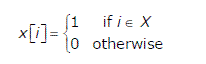# GATE | GATE-CS-2006 | Question 50

A set X can be represented by an array x[n] as follows:Consider the following algorithm in which x,y and z are Boolean arrays of size n:

 `algorithm zzz(x[] , y[], z []) ` `{ ` `   ``int` `i; ` `   ``for` `(i=O; i

The set Z computed by the algorithm is:
(A) (X ∩ Y)
(B) (X ∪ Y)
(C) (X-Y) ∩ (Y-X)
(D) (X-Y) ∪ (Y-X)